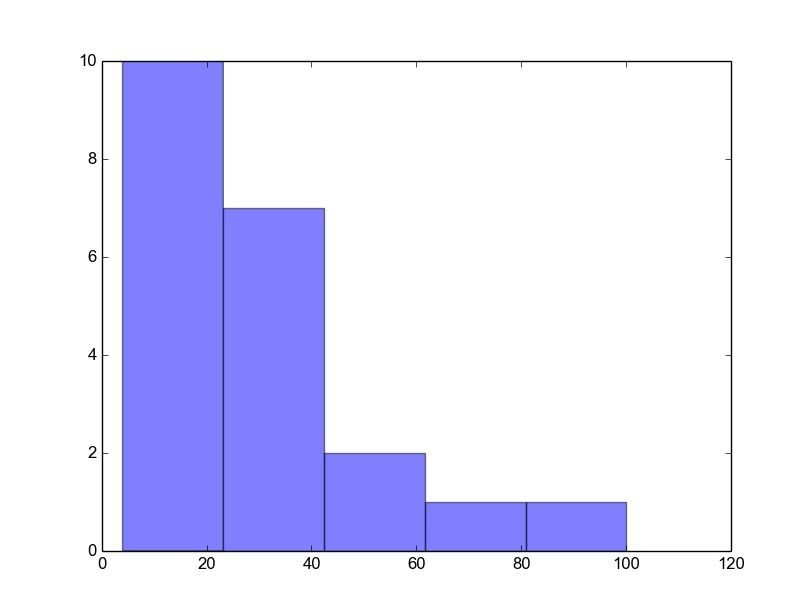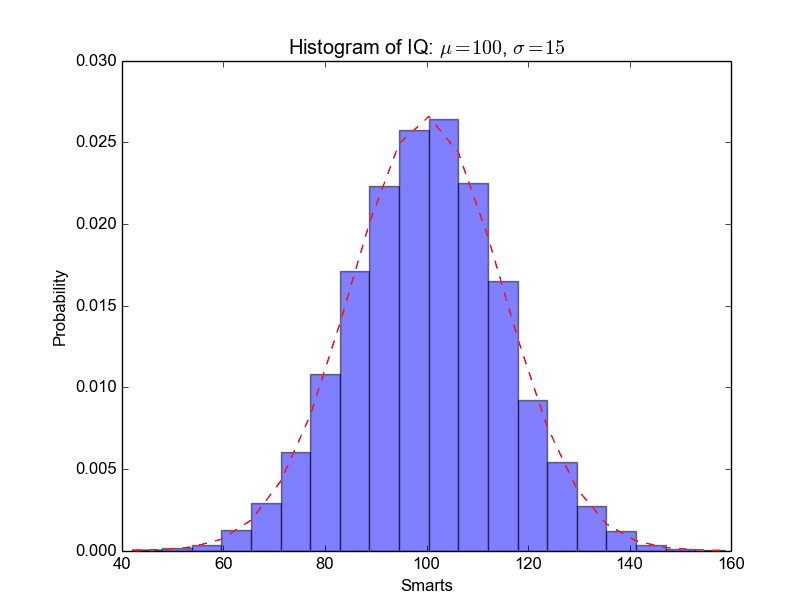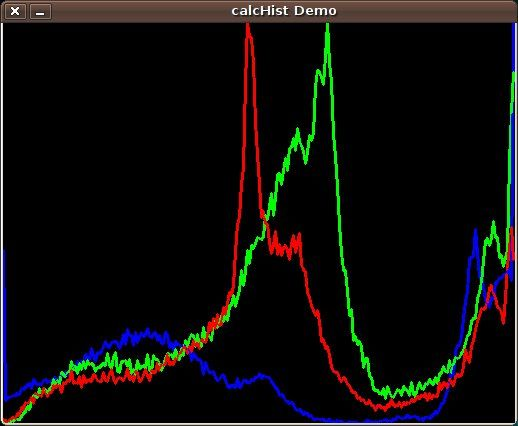# Tag: histogram

## Matplotlib Histogram

Matplotlib can be used to create histograms. A histogram shows the frequency on the vertical axis and the horizontal axis is another dimension. Usually it has bins, where every bin has a minimum and maximum value. Each bin also has a frequency between x and infinite.

Related course

Matplotlib histogram example
Below we show the most minimal Matplotlib histogram:

Output:Python histogram

A complete matplotlib python histogram
Many things can be added to a histogram such as a fit line, labels and so on. The code below creates a more advanced histogram.

Output:python_histogram

## Image histogram

A histogram is collected counts of data organized into a set of bins. Every bin shows the frequency. OpenCV can generate histograms for both color and gray scale images. You may want to use histograms for computer vision tasks.

Related course: Master Computer Vision with OpenCV

Histogram example
Given an image we can generate a histogram for the blue, green and red values.Histogram Calculation

We use the function cv.CalcHist(image, channel, mask, histSize, range)

Parameters:

• image:  should be in brackets,  the source image of type uint8 or float32
• channel:  the color channel to select. for grayscale use . color image has blue, green and red channels
• mask:  None if you want a histogram of the full image, otherwise a region.
• histSize:  the number of bins
• range:  color range:

Histogram for a color image:

Related course: Master Computer Vision with OpenCV